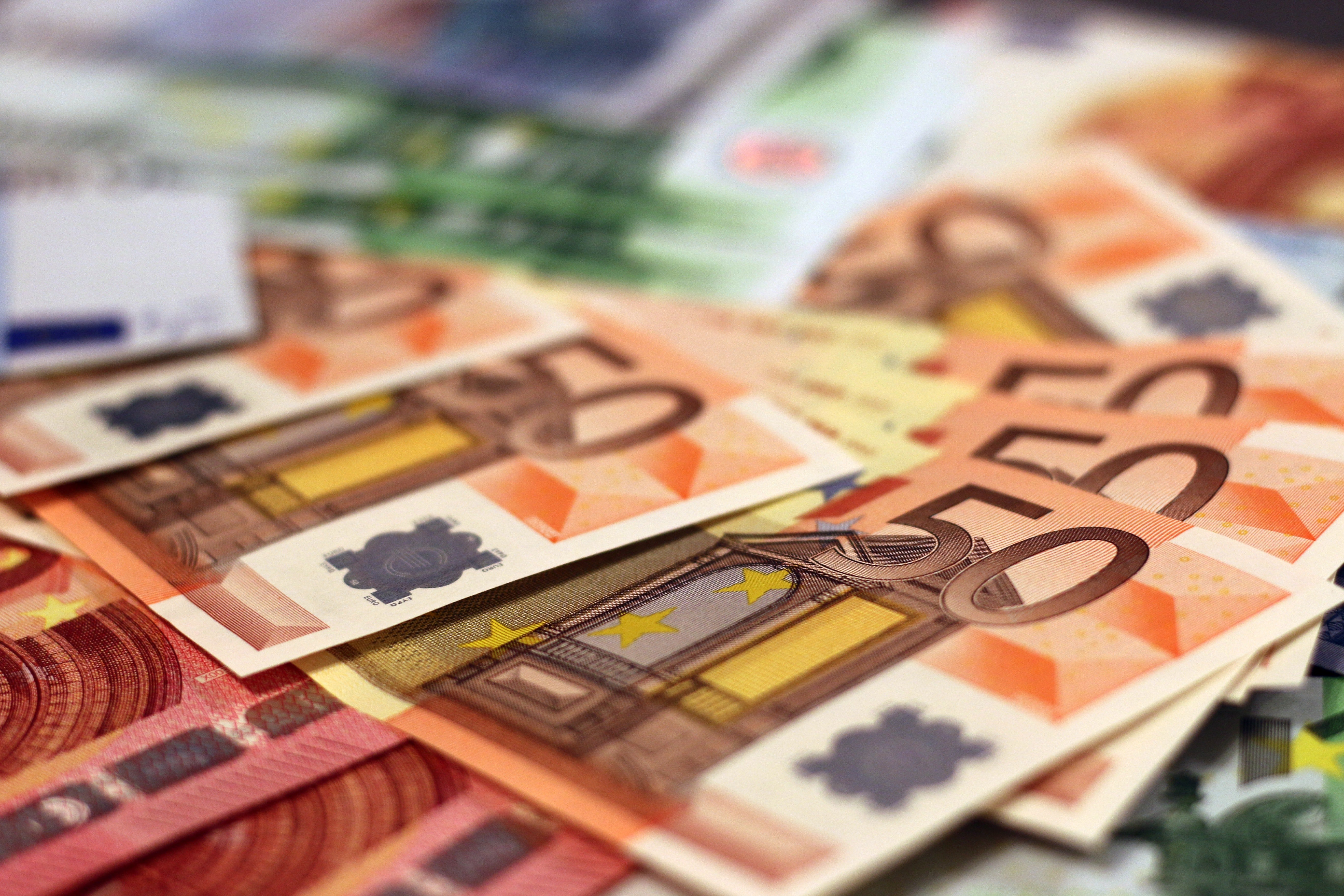﻿ Strategy Evaluation using the Sharpe and Sortino Ratios - FXCM UK# Strategy Evaluation using the Sharpe and Sortino Ratios

Successful traders approach trading with a clearly defined and thoroughly tested strategy. Most traders evaluate the hypothetical future performance of their strategy by measuring the profit or loss of the strategy run on historical data. But is measuring historical profitability enough? Historical profitability provides only a small piece of information about a trading strategy, while another factor that may be important to a trader is the riskiness of their strategy. The Sharpe Ratio and the Sortino Ratio are two methods of evaluating the risk of a strategy by comparing the returns to that of a risk-free investment. This article from QuantNews further explains both of these metrics and provides the formulas used to calculate them.

## The Sharpe Ratio

The Sharpe Ratio is calculated by dividing a strategy's excess return beyond the risk-free rate of return by the standard deviation of the returns. Generally measurements above 1 are considered preferable; the higher the better, as this would indicate the returns are achieved with limited volatility of the account equity.

## The Sortino Ratio

The Sortino Ratio is a variation of the Sharpe ratio that removes upside volatility from the calculation by considering the standard deviation of the returns to the downside. The Sharpe Ratio considers the standard deviation of both positive and negative returns, so a strategy with extreme positive returns would be penalized the same way as a strategy with extreme negative returns. For s strategy intended to provide consistent returns, the Sharpe Ratio would be an important measurement. However, for a strategy without this limitation, the Sortino Ratio may be a better way to measure risk-adjusted returns.

Whether you are backtesting a strategy or evaluating historical returns of a strategy, the Sharpe and Sortino Ratios can be useful metrics of risk in relation to returns. For those using a Python-based backtesting or live trading system, the article also includes sample Python code for calculating these ratios.